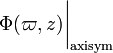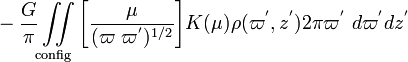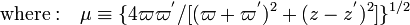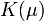# Template:LSU CT99CommonTheme1

The gravitational potential (both inside and outside) of any axisymmetric mass distribution may be determined from the following integral expression that we will refer to as the,

Gravitational Potential of an Axisymmetric Mass Distribution (Version 1)$~\Phi(\varpi,z)\biggr|_\mathrm{axisym}$$~=$$~ - \frac{G}{\pi} \iint\limits_\mathrm{config} \biggl[ \frac{\mu}{(\varpi~ \varpi^')^{1 / 2}} \biggr] K(\mu) \rho(\varpi^', z^') 2\pi \varpi^'~ d\varpi^' dz^'$$\mathrm{where:}~~~\mu \equiv \{4\varpi \varpi^' /[ (\varpi+\varpi^')^2 + (z-z^')^2]\}^{1 / 2}$

and,$~K(\mu)$ is the complete elliptic integral of the first kind. This Key Equation may be straightforwardly obtained, for example, by combining Eqs. (31), (32b), and (24) from Cohl & Tohline (1999); see also, Bannikova et al. (2011), Trova, Huré & Hersant (2012), and Fukushima (2016).

------------------------------

Building upon a previously little-known Compact Cylindrical Green's Function expansion, Cohl & Tohline (1999) derived an integral expression for the gravitational potential that is applicable to all mass distributions, irrespective of geometric symmetries. The Key Equation highlighted here — that is relevant to axisymmetric mass distributions — is a special case of this more general expression.